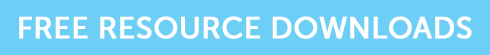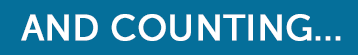How to Calculate Redemption and Running Yields on a Bond

# How to Calculate Redemption and Running Yields on a Bond

If you are sitting a CII investment exam, then we can almost guarantee that you will have to work out the yield on a bond and probably both the running yield as well as the redemption yield. So here’s a quick reminder of how to do it.

### Running Yield

Also known as the flat yield or income yield, running yield is simply measuring the annual income being received as a percentage of the price paid – in other words what you are getting back as a percentage of what you gave. The formula is:So if a bond is priced at a clean price of £120 and it’s paying a coupon of 5% then you divide 5% by £120 to get 0.04166 – multiply this by 100 to get 4.17%.

The investor actually receives £5 gross each year, but because the price paid was more than £100 (it was trading above par), then the equivalent return is less than 5%.

To take this one step further, we need to remember that if this bond is held until redemption, the investor will only get back £100 so will make a loss.

### Redemption Yield

So let’s now calculate the redemption yield assuming the investor does hold it until maturity.

Let’s assume the bond has 6 years left to run.

It was bought for £120, so at maturity there will be a capital loss of £20. With 6 years left to run, we can say that this is a £3.33 capital loss for each year left. As a percentage of the price that was paid this is – 2.78%. We then take this from the running yield we worked out earlier of 4.17% to give an approximate yield to redemption of 1.39%.

The formula is:Anyone taking a CII investment exam would be wise to practise these two calculations.

### Grab the resources you need!

If you’re studying for your CII R02 exam, and you think you need to practise some more, grab our free taster to try out one of Brand Financial Training’s resources for yourself.  Click the link to download the R02 mock paper taster now!Alternatively, you can download the taster for R06, AF4, AF5, J10 or J12 if one of those exams is causing you some anxiety.

### Over to You…

So do you think you would be able to calculate the returns on a bond in an exam situation? If this helped you, please let us know in the comments – we’d love to hear from you!